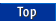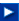Apache C++ Standard Library User's Guide

## 13.5 Removal Algorithms

The std::remove() algorithm and the std::unique() algorithm can be somewhat confusing the first time you encounter them. Both claim to remove certain values from a sequence, yet neither reduces the size of the sequence. Both operate by moving the values that are to be retained to the front of the sequence, and returning an iterator that describes where this sequence ends. Elements after this iterator are simply the original sequence values, left unchanged. This is necessary because the generic algorithm has no knowledge of the container it is working on. It only has a generic iterator. This is part of the price we pay for generic algorithms. In most cases you will want to use this iterator result as an argument to the std::erase() member function for the container, removing the values from the iterator to the end of the sequence.

Let us illustrate this with a simple example. Suppose we want to remove the even numbers from the sequence 1 2 3 4 5 6 7 8 9 10, something we could do with the std::remove_if() algorithm. Applying this algorithm would leave us with the following sequence:

1 3 5 7 9 | 6 7 8 9 10

The vertical bar here represents the position of the iterator returned by the std::remove_if() algorithm. Notice that the five elements before the bar represent the result we want, while the five values after the bar are simply the original contents of those locations. Using this iterator value along with the end-of-sequence iterator as arguments to std::erase(), we can eliminate the unwanted values, and obtain the desired result.

Both the algorithms described here have an alternative copy version. The copy version of the algorithms leaves the original unchanged, and places the preserved elements into an output sequence.

NOTE -- The example functions described in this section can be found in the file alg4.cpp.

### 13.5.1 Remove Unwanted Elements

The algorithm std::remove() eliminates unwanted values from a sequence. As with the std::find() algorithm, these can be values that match a specific constant, or values that satisfy a given predicate. The declaration of the argument types is as follows:

```namespace std {
ForwardIterator remove(ForwardIterator first,
ForwardIterator last, const T&);
ForwardIterator remove_if(ForwardIterator first,
ForwardIterator last, Predicate);
}
```

The algorithm std::remove() copies values to the front of the sequence, overwriting the location of the removed elements. All elements not removed remain in their relative order. Once all values have been examined, the remainder of the sequence is left unchanged. The iterator returned as the result of the operation provides the end of the new sequence. For example, eliminating the element 2 from the sequence 1 2 4 3 2 results in the sequence 1 4 3 3 2, with the iterator returned as the result pointing at the second 3. This value can be used as argument to std::erase() in order to eliminate the remaining elements 3 and 2, as illustrated in the example program.

A copy version of the algorithms copies values to an output sequence, rather than making transformations in place.

```namespace std {
OutputIterator remove_copy
(InputIterator first, InputIterator last,
OutputIterator result, const T&);

OutputIterator remove_copy_if
(InputIterator first, InputIterator last,
OutputIterator result, Predicate);
}
```

The use of std::remove() is shown in the following program.

```void remove_example()
// illustrates the use of the remove algorithm
// see alg4.cpp for complete source code
{
// create a list of numbers
int data[] = {1, 2, 4, 3, 1, 4, 2};
std::list<int> aList;
std::copy(data, data+7, std::inserter(aList, aList.begin()));

// remove 2's, copy into new list
std::list<int> newList;
std::remove_copy(aList.begin(), aList.end(),
std::back_inserter(newList), 2);

// remove 2's in place
std::list<int>::iterator where;
where = std::remove(aList.begin(), aList.end(), 2);
aList.erase(where, aList.end());

// remove all even values
where = std::remove_if(aList.begin(), aList.end(), isEven);
aList.erase(where, aList.end());
}
```

### 13.5.2 Remove Runs of Similar Values

The algorithm std::unique() moves through a linear sequence, eliminating all but the first element from every consecutive group of equal elements. The argument sequence is described by forward iterators:

```namespace std {
ForwardIterator unique (ForwardIterator first,
ForwardIterator last [, BinaryPredicate ] );
}
```

As the algorithm moves through the collection, elements are moved to the front of the sequence, overwriting the existing elements. Once all unique values are identified, the remainder of the sequence is left unchanged. For example, a sequence such as 1 3 3 2 2 2 4 is changed into 1 3 2 4 | 2 2 4. We use a vertical bar to indicate the location returned by the iterator result value. This location marks the end of the unique sequence, and the beginning of the left-over elements. With most containers, the value returned by the algorithm can be used as an argument in a subsequent call on std::erase() to remove the undesired elements from the collection. This is illustrated in the example program.

A copy version of the algorithm moves the unique values to an output iterator, rather than making modifications in place. In transforming a list or multiset, an insert iterator can be used to change the copy operations of the output iterator into insertions.

```namespace std {
OutputIterator unique_copy(InputIterator first,
InputIterator last,
OutputIterator result
[, BinaryPredicate ] );
}
```

These are illustrated in the sample program:

```void unique_example()
// illustrates the use of the unique algorithm
// see alg4.cpp for complete source code
{
// first make a list of values
int data[] = {1, 3, 3, 2, 2, 4};
std::list<int> aList;
std::set<int> aSet;
std::copy(data, data+6, std::inserter(aList, aList.begin()));

// copy unique elements into a set
std::unique_copy (aList.begin(), aList.end(),
std::inserter(aSet, aSet.begin()));

// copy unique elements in place
std::list<int>::iterator where;
where = std::unique(aList.begin(), aList.end());

// remove trailing values
aList.erase(where, aList.end());
}
```